# Airplane Image Classification using a Keras CNN

## Data Preprocessing

`# Importsimport globimport numpy as npimport os.path as pathfrom scipy import misc`
`# IMAGE_PATH should be the path to the downloaded planesnet folderIMAGE_PATH = ''file_paths = glob.glob(path.join(IMAGE_PATH, '*.png'))`
`# Load the imagesimages = [misc.imread(path) for path in file_paths]images = np.asarray(images)`
`# Get image sizeimage_size = np.asarray([images.shape, images.shape, images.shape])print(image_size)`
`# Scaleimages = images / 255`
`# Read the labels from the filenamesn_images = images.shapelabels = np.zeros(n_images)for i in range(n_images):    filename = path.basename(file_paths[i])    labels[i] = int(filename)`
`# Split into test and training setsTRAIN_TEST_SPLIT = 0.9# Split at the given indexsplit_index = int(TRAIN_TEST_SPLIT * n_images)shuffled_indices = np.random.permutation(n_images)train_indices = shuffled_indices[0:split_index]test_indices = shuffled_indices[split_index:]# Split the images and the labelsx_train = images[train_indices, :, :, :]y_train = labels[train_indices]x_test = images[test_indices, :, :, :]y_test = labels[test_indices]`

## Data Visualization

`import matplotlib.pyplot as plt`
`def visualize_data(positive_images, negative_images):    # INPUTS    # positive_images - Images where the label = 1 (True)    # negative_images - Images where the label = 0 (False)    figure = plt.figure()    count = 0    for i in range(positive_images.shape):        count += 1        figure.add_subplot(2, positive_images.shape, count)        plt.imshow(positive_images[i, :, :])        plt.axis('off')        plt.title("1")        figure.add_subplot(1, negative_images.shape, count)        plt.imshow(negative_images[i, :, :])        plt.axis('off')        plt.title("0")    plt.show()`
`# Number of positive and negative examples to showN_TO_VISUALIZE = 10# Select the first N positive examplespositive_example_indices = (y_train == 1)positive_examples = x_train[positive_example_indices, :, :]positive_examples = positive_examples[0:N_TO_VISUALIZE, :, :]# Select the first N negative examplesnegative_example_indices = (y_train == 0)negative_examples = x_train[negative_example_indices, :, :]negative_examples = negative_examples[0:N_TO_VISUALIZE, :, :]# Call the visualization functionvisualize_data(positive_examples, negative_examples)`
`# Importsfrom keras.models import Sequentialfrom keras.layers import Activation, Dropout, Flatten, Dense, Conv2D, MaxPooling2Dfrom keras.callbacks import EarlyStopping, TensorBoardfrom sklearn.metrics import accuracy_score, f1_scorefrom datetime import datetime`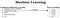A poorly drawn figure describing how deep learning fits in the broader ML world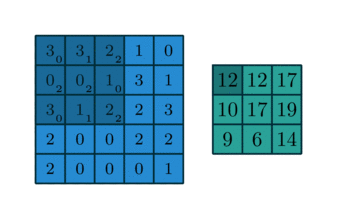Convolution of an image (Courtesy of: http://deeplearning.net/software/theano/_images/numerical_no_padding_no_strides.gif)
`# HyperparamaterN_LAYERS = 4`
`def cnn(size, n_layers):    # INPUTS    # size     - size of the input images    # n_layers - number of layers    # OUTPUTS    # model    - compiled CNN    # Define hyperparamters    MIN_NEURONS = 20    MAX_NEURONS = 120    KERNEL = (3, 3)    # Determine the # of neurons in each convolutional layer    steps = np.floor(MAX_NEURONS / (n_layers + 1))    nuerons = np.arange(MIN_NEURONS, MAX_NEURONS, steps)    nuerons = nuerons.astype(np.int32)    # Define a model    model = Sequential()    # Add convolutional layers    for i in range(0, n_layers):        if i == 0:            shape = (size, size, size)            model.add(Conv2D(nuerons[i], KERNEL, input_shape=shape))        else:            model.add(Conv2D(nuerons[i], KERNEL))        model.add(Activation('relu'))    # Add max pooling layer    model.add(MaxPooling2D(pool_size=(2, 2)))    model.add(Flatten())    model.add(Dense(MAX_NEURONS))    model.add(Activation('relu'))    # Add output layer    model.add(Dense(1))    model.add(Activation('sigmoid'))    # Compile the model    model.compile(loss='binary_crossentropy',                  optimizer='adam',                  metrics=['accuracy'])    # Print a summary of the model    model.summary()    return model`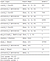Summary of model architecture
`# Instantiate the modelmodel = cnn(size=image_size, n_layers=N_LAYERS)`
`# Training hyperparamtersEPOCHS = 150BATCH_SIZE = 200`
`# Early stopping callbackPATIENCE = 10early_stopping = EarlyStopping(monitor='loss', min_delta=0, patience=PATIENCE, verbose=0, mode='auto')`
`# TensorBoard callbackLOG_DIRECTORY_ROOT = ''now = datetime.utcnow().strftime("%Y%m%d%H%M%S")log_dir = "{}/run-{}/".format(LOG_DIRECTORY_ROOT, now)tensorboard = TensorBoard(log_dir=log_dir, write_graph=True, write_images=True)`
`tensorboard --logdir LOG_DIRECTORY_ROOT`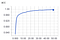Accuracy vs. training iteration
`# Place the callbacks in a listcallbacks = [early_stopping, tensorboard]`
`# Train the modelmodel.fit(x_train, y_train, epochs=EPOCHS, batch_size=BATCH_SIZE, callbacks=callbacks, verbose=0)`
`# Make a prediction on the test settest_predictions = model.predict(x_test)test_predictions = np.round(test_predictions)`
`# Report the accuracyaccuracy = accuracy_score(y_test, test_predictions)print("Accuracy: " + str(accuracy))`
`import matplotlib.pyplot as pltdef visualize_incorrect_labels(x_data, y_real, y_predicted):    # INPUTS    # x_data      - images    # y_data      - ground truth labels    # y_predicted - predicted label    count = 0    figure = plt.figure()    incorrect_label_indices = (y_real != y_predicted)    y_real = y_real[incorrect_label_indices]    y_predicted = y_predicted[incorrect_label_indices]    x_data = x_data[incorrect_label_indices, :, :, :]    maximum_square = np.ceil(np.sqrt(x_data.shape))    for i in range(x_data.shape):        count += 1        figure.add_subplot(maximum_square, maximum_square, count)        plt.imshow(x_data[i, :, :, :])        plt.axis('off')        plt.title("Predicted: " + str(int(y_predicted[i])) + ", Real: " + str(int(y_real[i])), fontsize=10)    plt.show()visualize_incorrect_labels(x_test, y_test, np.asarray(test_predictions).ravel())`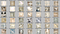Output showing all of the false positives and false negatives in the test dataset

Written by

## Kyle O'Brien

#### I am a Computer Vision Engineer working in NYC

Welcome to a place where words matter. On Medium, smart voices and original ideas take center stage - with no ads in sight. Watch
Follow all the topics you care about, and we’ll deliver the best stories for you to your homepage and inbox. Explore
Get unlimited access to the best stories on Medium — and support writers while you’re at it. Just \$5/month. Upgrade